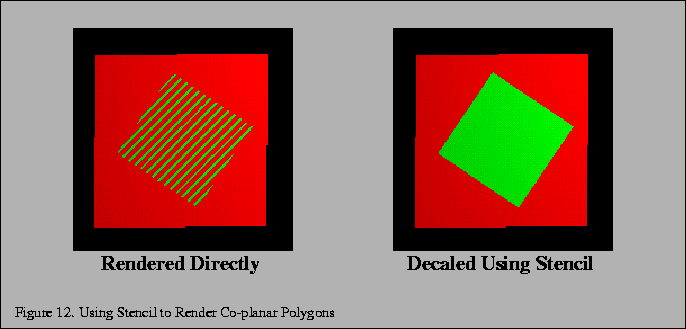Next: 3.6 Capping Clipped Solids Up: 3. Modeling Previous: 3.4.1 Greedy Tri-stripping

## 3.5 Coplanar Polygons and Decaling with Stencil

Using stenciling to control pixels drawn from a particular primitive can help solve a number of important problems:

1.
Drawing depth-buffered, co-planar polygons without z-buffering artifacts.
2.
Decaling multiple textures on a primitive.

Values are written to the stencil buffer to create a mask for area to be decaled. Then this stencil mask is used to control two separate draw steps; one for the decaled region, one for the rest of the polygon.

A useful example that illustrates the technique is rendering co-planar polygons. If one polygon is to be rendered directly on top of another (runway markings, for example), the depth buffer ca not be relied upon to produce a clean separation between the two. This is due to the quantization of the depth buffer. Since the polygons have different vertices, the rendering algorithms can produce z values that are rounded to the wrong depth buffer value, so some pixels of the back polygon may show through the front polygon. In an application with a high frame rate, this results in a shimmering mixture of pixels from both polygons (commonly called Z fighting'' or flimmering''). An example is shown in in Figure 12.To solve this problem, the closer polygons are drawn with the depth test disabled, on the same pixels covered by the farthest polygons. It appears that the closer polygons are decaled'' on the farther polygons.

Decaled polygons can be drawn with the following steps:

1.
Turn on stenciling; glEnableGL_STENCIL_TEST(GL_STENCIL_TEST).
2.
Set stencil function to always pass; glStencilFuncGL_ALWAYS, 1, 1(GL_ALWAYS, 1, 1).
3.
Set stencil op to set 1 if depth passes, 0 if it fails; glStencilOpGL_KEEP, GL_ZERO, GL_REPLACE(GL_KEEP, GL_ZERO, GL_REPLACE).
4.
Draw the base polygon.
5.
Set stencil function to pass when stencil is 1; glStencilFuncGL_EQUAL, 1, 1(GL_EQUAL, 1, 1).
6.
Disable writes to stencil buffer; glStencilMaskGL_FALSE(GL_FALSE).
7.
Turn off depth buffering; glDisableGL_DEPTH_TEST(GL_DEPTH_TEST).
8.
Render the decal polygon.

The stencil buffer does not have to be cleared to an initial value; the stencil values are initialized as a side effect of writing the base polygon. Stencil values will be one where the base polygon was successfully written into the framebuffer, and zero where the base polygon generated fragments that failed the depth test. The stencil buffer becomes a mask, ensuring that the decal polygon can only affect the pixels that were touched by the base polygon. This is important if there are other primitives partially obscuring the base polygon and decal polygons.

There are a few limitations to this technique. First, it assumes that the decal polygon does not extend beyond the edge of the base polygon. If it does, you will have to clear the entire stencil buffer before drawing the base polygon, which is expensive on some machines. If you are careful to redraw the base polygon with the stencil operations set to zero the stencil after you've drawn each decaled polygon, you will only have to clear the entire stencil buffer once, for any number of decaled polygons.

Second, if the screen extents of the base polygons you're decaling overlap, you will have to perform the decal process for one base polygon and its decals before you move on to another base and decals. This is an important consideration if your application collects and then sorts geometry based on its graphics state, where the rendering order of geometry may be changed by the sort.

This process can be extended to allow a number of overlapping decal polygons, the number of decals limited by the number of stencil bits available for the visual. The decals do not have to be sorted. The procedure is the similar to the previous algorithm, with the following extensions.

Assign a stencil bit for each decal and the base polygon. The lower the number, the higher the priority of the polygon. Render the base polygon as before, except instead of setting its stencil value to one, set it to the largest priority number. For example, if there were three decal layers, the base polygon would have a value of 8.

When you render a decal polygon, only draw it if the decal's priority number is lower than the pixels it is trying to change. For example, if the decal's priority number was 1, it would be able to draw over every other decal and the base polygon; glStencilFuncGL_LESS, 1,  0(GL_LESS, 1,  0) and glStencilOpGL_KEEP, GL_REPLACE, GL_REPLACE(GL_KEEP, GL_REPLACE, GL_REPLACE).

Decals with the lower priority numbers will be drawn on top of decals with higher ones. Since the region not covered by the base polygon is zero, no decals can write to it. You can draw multiple decals at the same priority level. If you overlap them, however, the last one drawn will overlap the previous ones at the same priority level.

Multiple textures can be drawn onto a polygon with a similar technique. Instead of writing decal polygons, the same polygon is drawn with each subsequent texture and an alpha value to blend the old pixel color and the new pixel color together.Next: 3.6 Capping Clipped Solids Up: 3. Modeling Previous: 3.4.1 Greedy Tri-stripping
David Blythe
1999-08-06